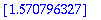Function: allsolve - find list of floating-point approximations to all roots of an equation or expression on a real interval.

Calling sequence:

allsolve( expr, x = a .. b);

Parameters:

expr - the equation or expression, involving one variable

x - the variable (a name)

a, b - endpoints of the interval (real constants).

Description:

allsolve computes numerically all the solutions of an equation expr in one variable x in the real interval a .. b . If expr is an expression rather than an equation, the equation expr = 0 is used. The result is returned as a list, sorted in numerical order.

Only one variable is allowed: the two sides of the equation must evaluate to real constants when any constant value in the interval a .. b is substituted for x .

The expression and all subexpressions should have at least two continuous derivatives on the interval. In particular, infinite limits at the endpoints, or indeterminate forms (such as f/g where f and g both approach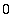or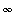at the endpoints) may cause trouble.

An exception to the requirements of continuity and differentiability is in the case of an expression defined piecewise, using piecewise , signum , Heaviside , abs , min or max , as long as convert(...,pwlist) can convert it to a list of expressions on different intervals. If this can't be done, an error occurs.

Infinite endpoints are allowed, but are not likely to work unless the expression has finite limits at those endpoints.

Since numerical techniques are used, the accuracy of the results is limited. In particular, a root of f(x) that is also a root of D(f)(x) may be computed with poor accuracy, or even missed entirely. In such a case it may be better to use D(f)(x) instead of f(x) . Increasing Digits should also improve accuracy.

In some difficult cases allsolve may take a very long time. In particular, this will happen if the function is complicated or changes direction rapidly in the interval.

allsolve uses evalr to do interval arithmetic, and is therefore subject to the weaknesses of that procedure. In particular, it doesn't work with the two-variable version of arctan .

This function is part of the Maple Advisor Database library, and must be loaded before use by the command readlib(allsolve); .

Examples:

> allsolve( tan(sin(x))=1, x = 0 .. 2*Pi );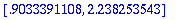> allsolve((x-Pi)^2, x = 0 .. 4);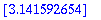> allsolve(sin(x) + x^2, x = -infinity .. infinity);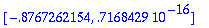> allsolve(abs(sin(x)+x) = 1, x = -2 .. 2);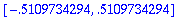> allsolve(Int(sin(s),s=0..x) = 1, x = 1 .. 2);